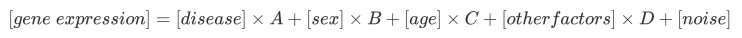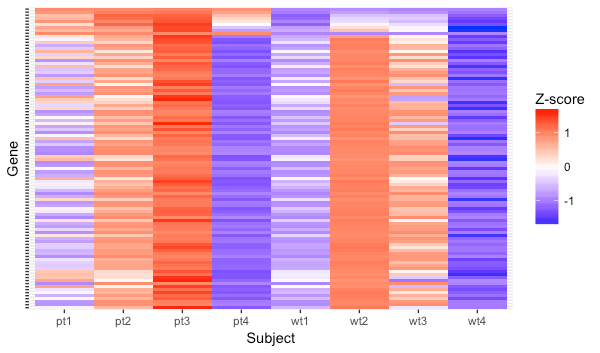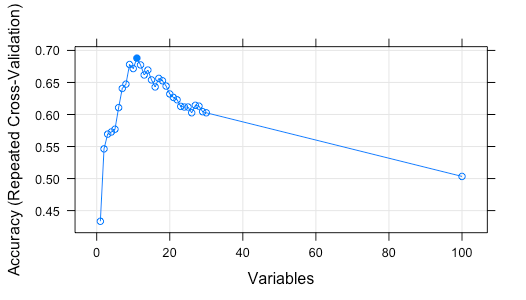# Seeking signal in the midst of noise with RPhoto by Martin Barák on Unsplash

Oftentimes, the sample I deal with is full of noise or confounding factors that I am not interested in. For example, human specimen is doomed noisy because the race, age, sex, occupation, or the life story of the subject would have influenced the results. Careful matching those statistics and increasing sample number would help a lot minimize known confounding factors and have a better chance to cancel other unknown factors, but sometimes sample number is just beyond our control.

I become curious about how well differential expression analysis works on this kind of dataset and whether other techniques could help us fish signal from noise. Thus, I made a dataset, in which 10 out of 100 “genes” are informative. In this dataset, there are 8 samples, with their disease state being either 0 (healthy) or 1 (diseased); sex being either 0 or 1; age being a number between 0–1, which represents age/100; and other factors is represented by a random number between 0–1.

`# Generating subject characteristics`
`# Disease statedisease <- c(0,0,0,0,1,1,1,1)`
`# Sexset.seed(1629)sex <- as.numeric(runif(n = 8, min = 0, max = 1) > 0.5)`
`# Hidden confounding factor for each Nset.seed(714)hcf <- runif(n = 8, min = 0, max = 1)`
`# Ageset.seed(2018)age <- round(runif(n = 8, min = 0, max = 1), digits = 2)`Simulating expression that correlates with multiple factors

Then, the expression of “genes” are calculated considering the age, sex, other confounding factors combined, and technical variation represented by a noise term that varies between 0–0.5.

In the equation above, A is 0.1 for gene #1, 0.2 for gene #2, and 1 for gene #10. A is 0 for the rest of the 90 genes. B, C, and D are randomly generated with the sum of A, B, C, and D restricted to < 1. So, only the first 10 genes are relevant to disease state, and gene #10 is the most representative one.

`# Saving the genes and the random generated coefficients in two listsfeature <- list()coef <- list()`
`# Generating 100 genesfor (i in seq(100)) {  set.seed(i)`
`# Generate 10 genes that is related to disease  if (i <= 10) {    coef_sex <- runif(n = 1, min = 0, max = (1 - i/10))    coef_hcf <- runif(n = 1, min = 0, max = (1 - i/10 - coef_sex))    coef_age <- 1 - (i/10 + coef_sex + coef_hcf)    noise <- runif(1, min = 0, max = 0.5)    feature[[i]] <- disease * (i / 10) + sex * coef_sex + age * coef_age + hcf * coef_hcf + noise    coef[[i]] <- c(i/10, coef_sex, coef_age, coef_hcf, noise)    next  }`
`# The rest 90 genes are no related to disease state  coef_sex <- runif(n = 1, min = 0, max = 1)  coef_hcf <- runif(n = 1, min = 0, max = (1 - coef_sex))  coef_age <- 1 - (coef_sex + coef_hcf)  noise <- runif(1, min = 0, max = 0.5)  feature[[i]] <- sex * coef_sex + age * coef_age + hcf * coef_hcf + noise  coef[[i]] <- c(0, coef_sex, coef_age, coef_hcf, noise)}`
`# Formatting the expression matrixexpression <- do.call("rbind", feature)coefficent <- do.call("rbind", coef)colnames(expression) <- c("wt1", "wt2", "wt3", "wt4", "pt1", "pt2", "pt3", "pt4")colnames(coefficent) <- c("Disease", "Sex", "Age", "HCF", "Noise")expression <- data.frame(expression)`
`expression\$gene <- c(sapply(seq(10), function(x) paste("real", x, sep = "_")),sapply(seq(90), function(x) paste("noise", x, sep = "_")))`The script above generated something like this.

From the heatmap, it seems that the other factors is contributing a lot to the difference between samples. Is differential expression analysis capable of finding the genes that are really related to diseases?

`library(limma)`
`log_exp <- log2(expression[, -9])log_exp\$gene <- expression\$gene`
`design <- model.matrix(~ 0+factor(c(0,0,0,0,1,1,1,1)))colnames(design) <- c("Ctrl","Patient")fit <- lmFit(log_exp[, -9], design)fit\$genes\$ID <- log_exp\$gene`
`cont.matrix <- makeContrasts(PatientvsCtrl = Patient-Ctrl, levels=design)fit2 <- contrasts.fit(fit, cont.matrix)fit2 <- eBayes(fit2)volcanoplot(fit2, highlight = 5)tt <- topTable(fit2, number = 20, adjust = "BH")`

It turned out `limma` did a great job. It hit 3 significantly up-regulated genes (real_10, real_9, and real_8), and from the volcano plot, we could see the disease correlated genes (annotated as “real_x”) are quite visible even if they were not significant.

Does fancier tools do a better job in finding real differentially expressed genes in a noisy dataset? I tried `caret` and `Boruta` to give machine learning a try.

`# Reverse feature selection with caretlibrary(caret)exp_rfe <- t(expression[, -9])colnames(exp_rfe) <- expression\$genectrl <- rfeControl(functions = rfFuncs,                   method = "repeatedCV",                   repeats = 10, verbose = TRUE)type_exp <- factor(c(0,0,0,0,1,1,1,1), labels = c("0", "1"))lmProfile_exp <- rfe(x=exp_rfe, y=type_exp,                     sizes = c(1:30),                     rfeControl = ctrl)plot(lmProfile_exp, type = c("g", "o"))predictors(lmProfile_exp)`

Reverse feature selection with `caret` gave me 4 genes (real_7–10), while `Boruta` rejected every single gene. It seemed that at least for this dataset, traditional differential expression performed at least as well as machine learning approaches.

Increase the noise term

Interestingly, when I gave noise term a coefficient of 10 instead of 1. `limma` identified more up-regulated genes (real_6–10), while the results from `caret` and `Boruta` remained the same. It was against my anticipation that the real difference would be buried in the noise, so I tried to use a coefficient of 10000. This time `limma` found even more up-regulated genes (real_4–10)…

Lower the effect size of disease state

On the other hand, When I made disease term 10 times smaller, `limma` could not find any significantly up-regulated gene, while `caret` managed to find one (real_9).

Increase sample number

Finally, to see whether sample number could improve sensitivity when disease term is small, I tried to do the same analysis on 80 subjects (40 control and 40 diseased). I was expecting both differential expression analysis and machine learning would benefit from a larger sample number.

With 80 samples, `limma` still could not find any significantly changed genes, `caret` found 5 (real_4, 6, 7, 8, 9) along with 5 false positive, and there was still no luck for `Boruta`.Reverse feature selection with caret (N = 80)

In this short experiment, I got a feeling that:

1. Effect size and consistency play the most important role in differential expression analysis. A small effect size gets overwhelmed by confounding factors and noise, while larger ones could be detected even with a large noise term.
2. With a small sample size, result of reverse feature selection with `caret` and `limma` are not much different than each other.
3. When effect size is small, increasing sample number helps a bit in `limma` (adjusted p value goes down with increased sample number). `caret` seems to benefit more from larger sample number (because it’s machine learning?), but even with more samples, false positive is quite concerning.

I guess the difficult choice between sensitivity and specificity would still be an issue here. Though I hoped machine learning could work like magical black box, it performed similar to traditional approaches. I would need to learn more about the basics of machine learning before I could tell if there is some better way doing this.